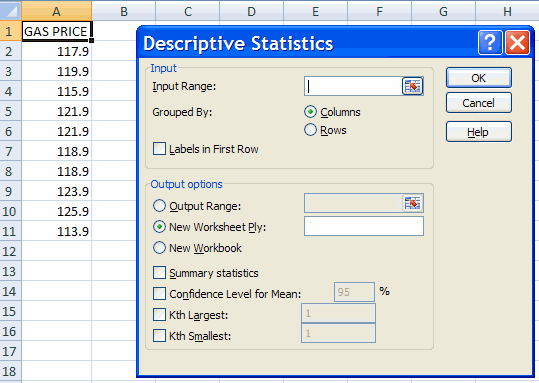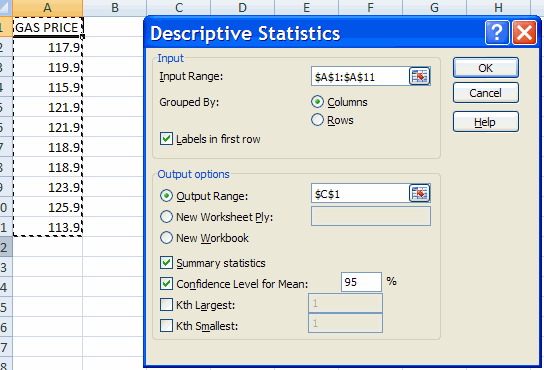## A. Colin Cameron, Dept. of Economics, Univ. of Calif. - Davis

This January 2009 help sheet gives information on how to obtain
• Descriptive statistics for data using Data Analysis Add-in
• Statistics using individual function commands.

DESCRIPTIVE STATISTICS USING DATA ANALYSIS ADD-IN

We consider the following gasoline price data (cents per gallon)
These data are in gasolinepricedata.csv

[Alternatively type in cells A1:A10 the ten values
117.9, 119.9, 115.9, 121.9, 121.9, 118.9, 118.9, 123.9, 125.9, 113.9.
and then insert a header GAS PRICE in new row 1] .

To obtain various descriptive statisticsClicking OK yields the dialog box• Choose input range as A1:A11 (by selecting this range with the mouse or by typing this in)
• Select labels in First rows (assuming labels have been provided)
• For output range choose e.g. cell C1 (choose a location where two adjacent columns have no data)
• Select summary statistics.
• Select confidence Level for mean (explained later under statistical inference).Selecting OK yields the outputAn explanation of the kwy parts of this output is the following:

 Mean 119.9 ybar = the sample mean. Standard error 1.14504 s/sqrt(n) = the estimated standard deviation of the sample mean Standard deviation 3.62093 s = the estimated standard deviation of y Variance 13.1111 s-squared = the estimated variance of y                  = Sum of (y_i - ybar)^2 Count 10 n = sample size Confidence level (95%) 2.59025 t(.025;n-1)*s/sqrt(n)

The mean is the simple average of the observations and is the standard estimate of the center of the data. (Other estimates are the median and the mode).
The standard deviation is the standard estimate of the variability of the data.
The standard error and the confidence level are used for statistical inference on the population mean and are discussed in Excel: Statistical Inference for univariate data

ADDITIONAL SUMMARY STATISTICS USING DATA ANALYSIS

The standard output gives Mean, Standard error, Median, Mean, Standard deviation, Variance, Kurtosis, Skewness, Range, Maximum, Minimum,
Sum, and Count.

Additionally one can obtain the k-th largest and k-th smallest values by checking the appropriate column and setting k.
For example, with 43 observations one might choose the 11th smallest and 11th largest to obtain approximate estimates of the lower quartile and upper quartile. (Easier is to separately use the Quartile or Percentile Commands).

STATISTICS BY INDIVIDUAL COMMAND

The descriptive statistics can be obtained separately by individual commands.
In addition one can find many more commands than appears in summary statistics.

Assume the data is in cells A2:A11. To obtain the sample mean and report it in, say, cell A13
either type in cell A13 the text = AVERAGE(A2:A11)
or do the following:

• Click on cell A13 (this is where the result will be stored).
• Select the Formulas Tab and the Function Library group
• Click on Autosum
• Choose function name AVERAGE
• Enter A2:A11.
You should have 119.9 in A13.

The following commands produce the same output as the default output for Tools | Data Analysis

• Mean:      = AVERAGE(A2:A11)
• Standard error:   = STDEV(A2:A11) / SQRT(COUNT(A2:A11))\
• Median:    = MEDIAN(A2:A11)
• Mean:      = MODE(A2:A11)
• Standard deviation:   = STDEV(A2:A11)
• Variance:   = VAR(A2:A11)
• Kurtosis:     = KURT(A2:A11)
• Skewness:   = SKEW(A2:A11)
• Range:       = MAX(A2:A11) - MIN(A2:A11)
• Maximum:   = MAX(A2:A11)
• Minimum:   = MIN(A2:A11)
• Sum:          = SUM(A2:A11)
• Count:        = COUNT(A2:A11)

The complete Excel 2007 statistical commands (in Formulas Tab | Function Library Group | More Functions | Statistical) are:

• AVEDEV, AVERAGE, AVERAGEA, AVERAGEIF, AVERAGEIFS
• CHIDIST, CHIINV, CHITEST, CONFIDENCE, CORREL, COUNT, COUNTA, COUNTBLANK, COUNTIF, COUNTIFS, COVAR, CRITBINOM
• DEVSQ
• EXPONDIST
• FDIST, FINV, FISHER, FISHERINV, FORECAST, FREQUENCY, FTEST
• GAMMADIST, GAMMAINV, GAMMLN, GEOMEAN, GROWTH
• HARMEAN, HYPGEOMDIST
• INTERCEPT
• KURT
• LARGE, LINEST, LOGEST, LOGINV, LOGNORMDIST
• MAX, MAXA, MEDIAN, MIN, MINA, MODE
• NEGBINOMDIST, NORMDIST, NORMINV, NORMSDIST, NORMSINV
• PEARSON, PERCENTILE, PRECENTRANK, PERMUT, POISSON, PROB
• QUARTILE
• RANK, RSQ
• SKEW, SLOPE, SMALL, STANDARDIZE, STDEV, STDEVA, STDEVP, STDEVPA, STEYX
• TDIST, TINV, TREND, TRIMMEAN, TTEST
• VAR, VARA, VARP, VARPA
• WEIBULL
• ZTEST.

For further information on how to use Excel go to
http://cameron.econ.ucdavis.edu/excel/excel.html